Successfully reported this slideshow.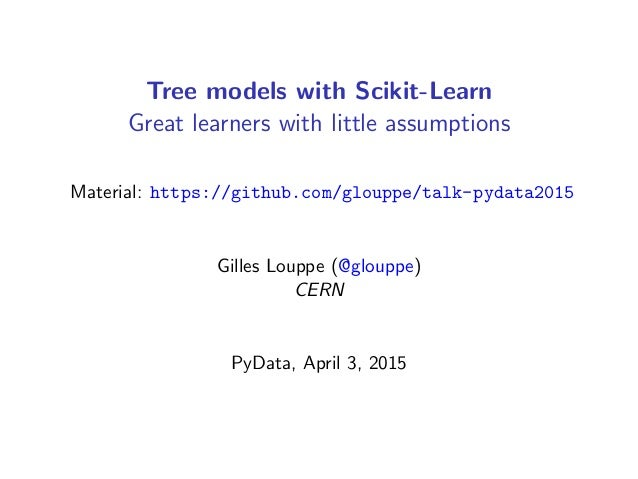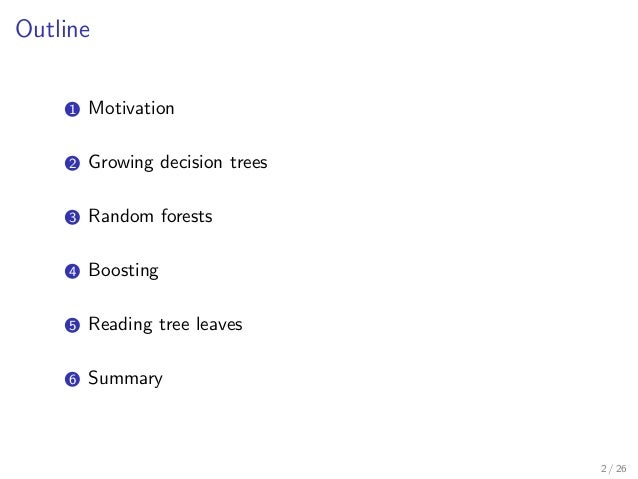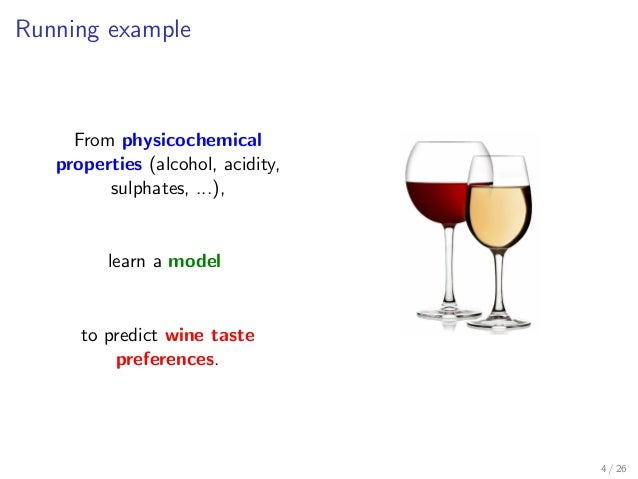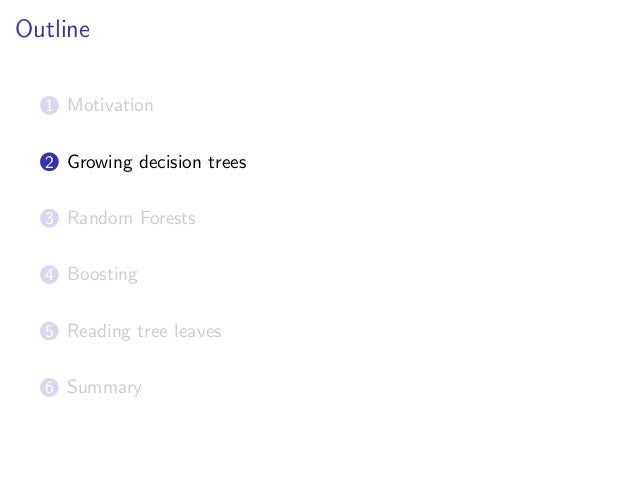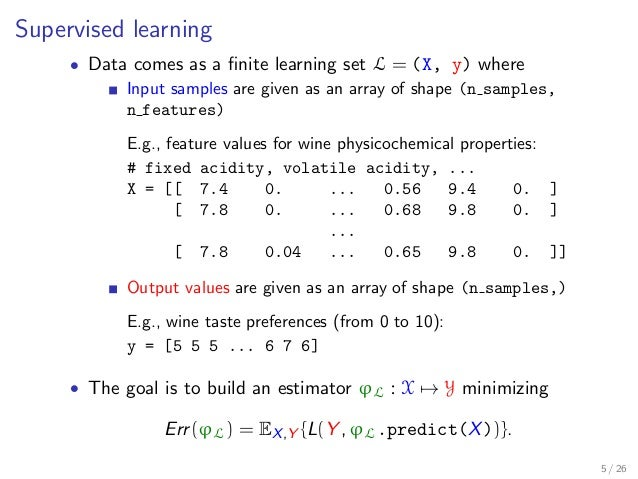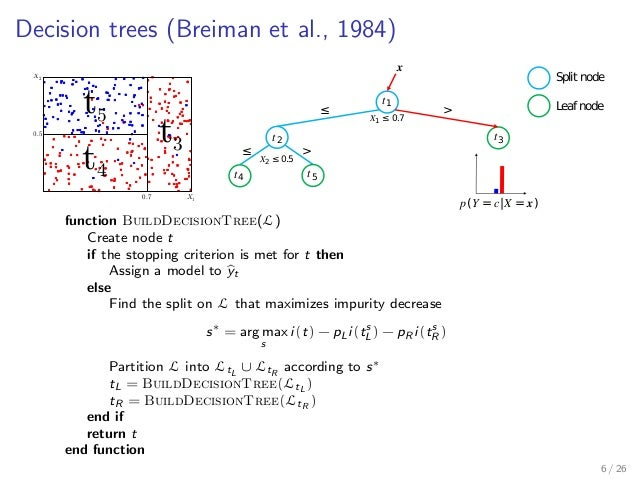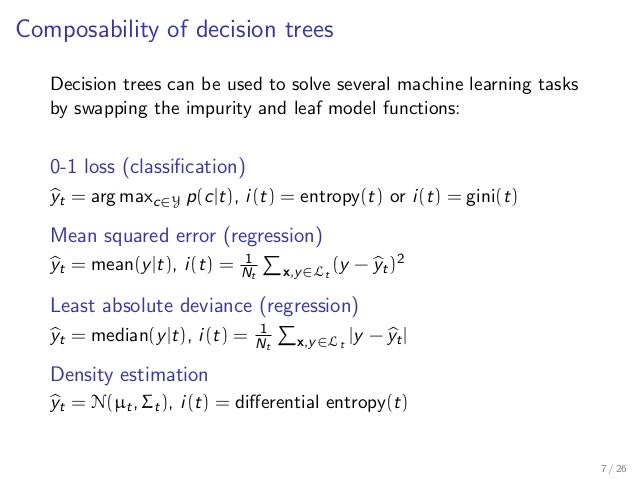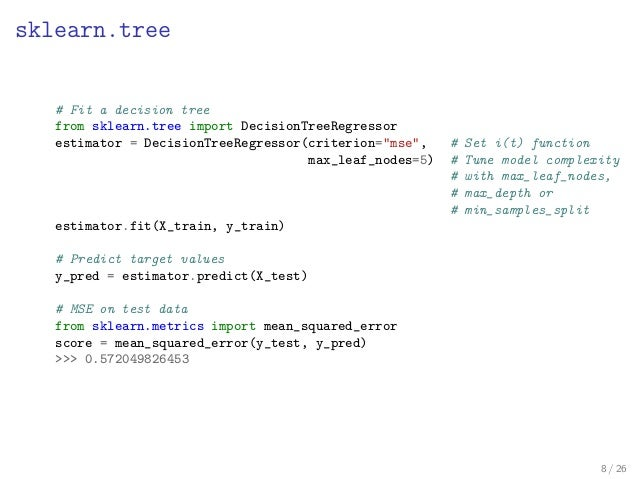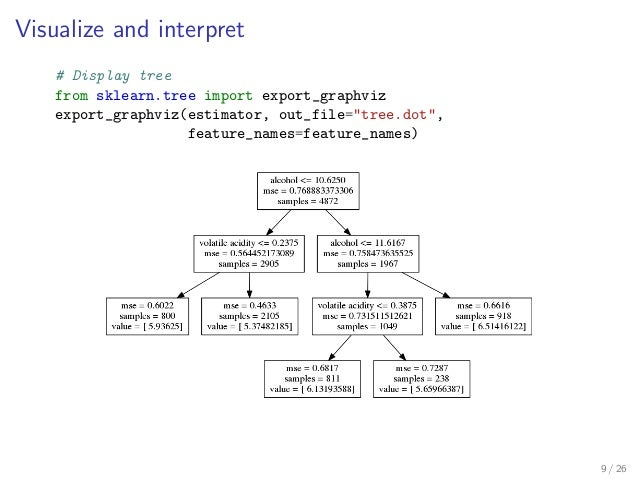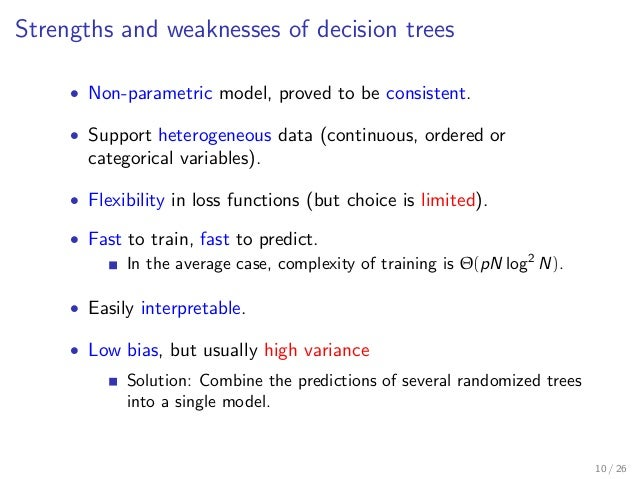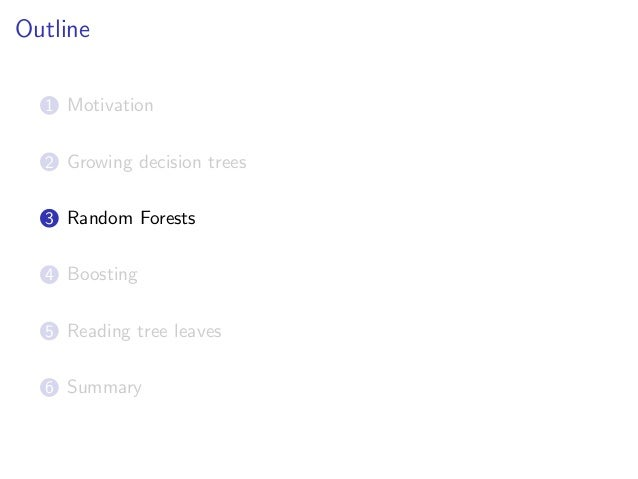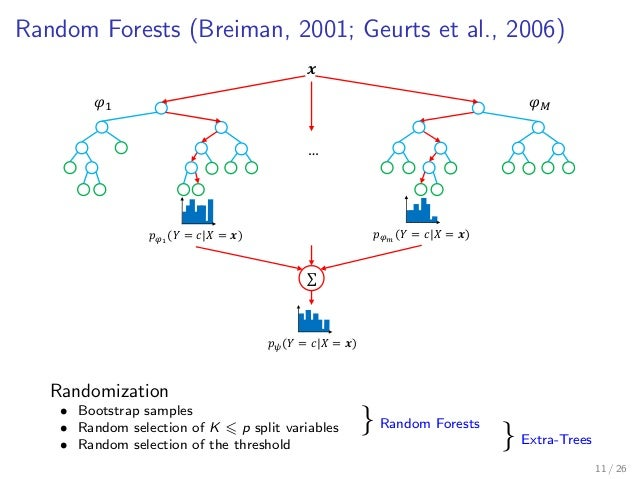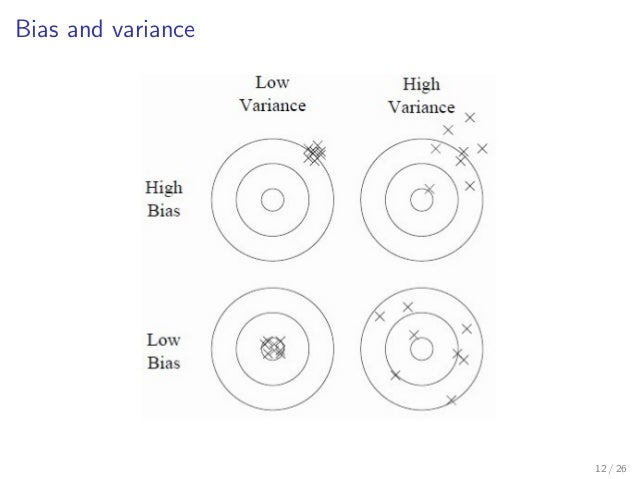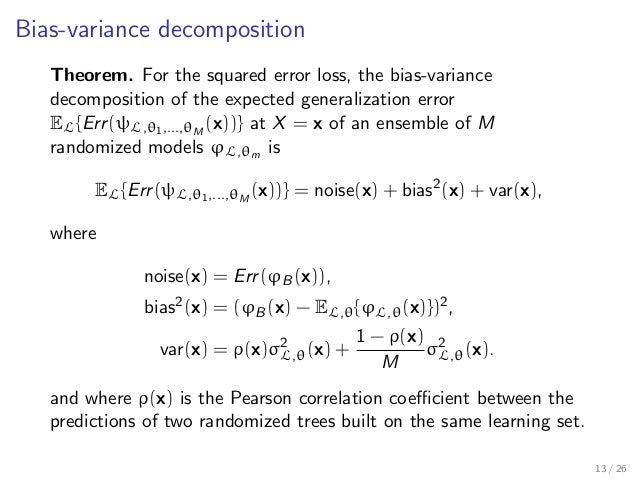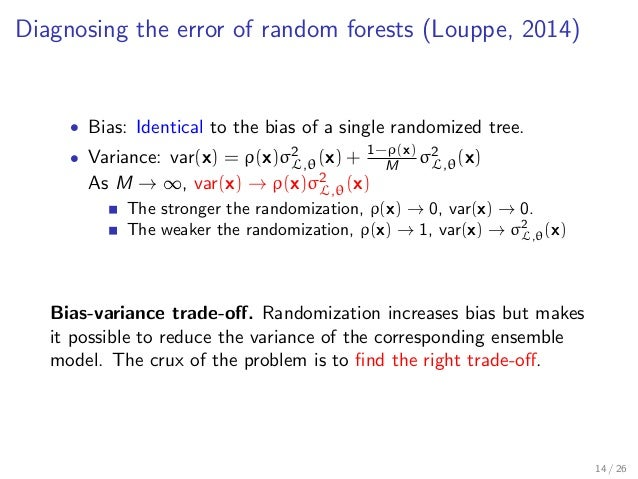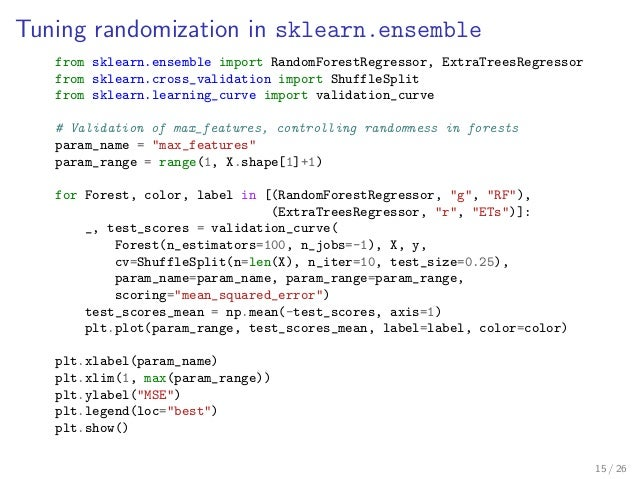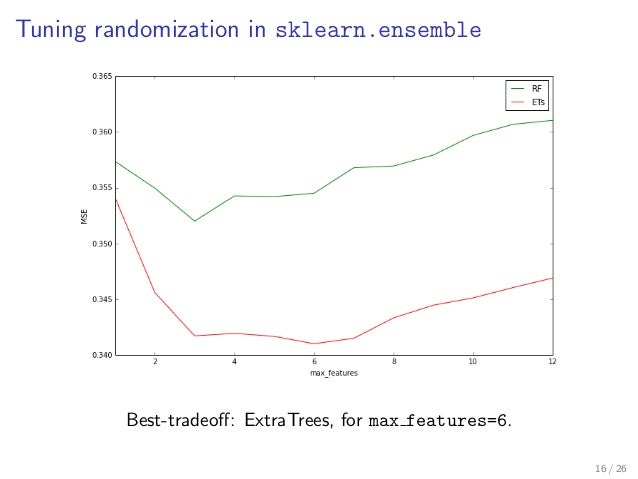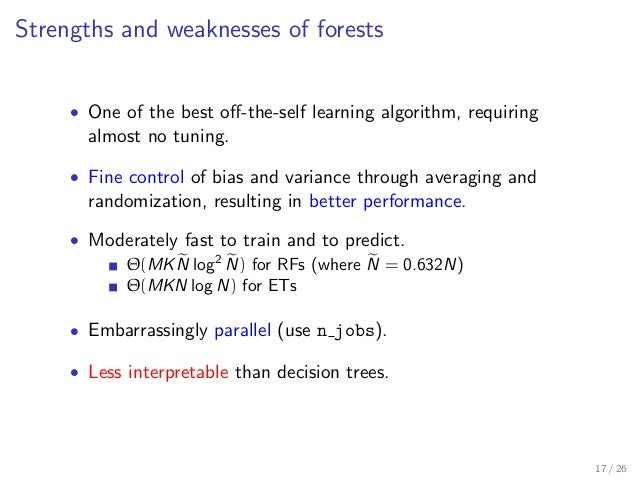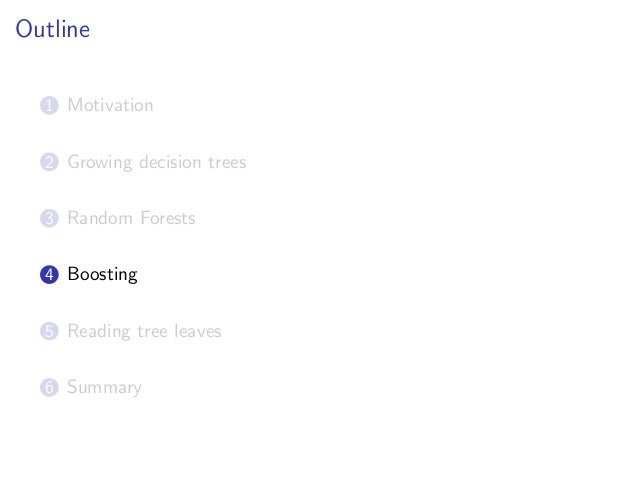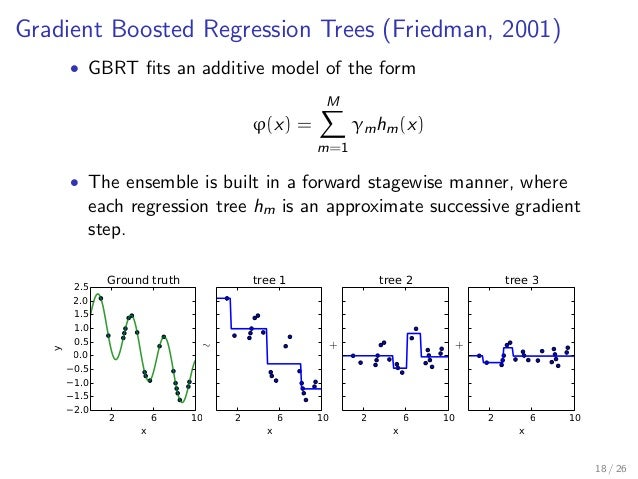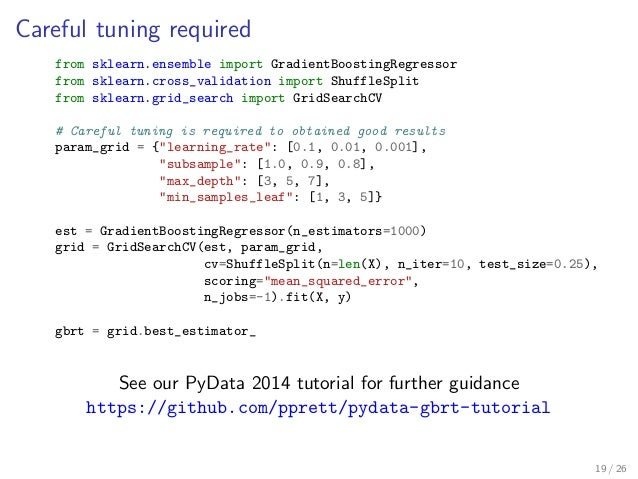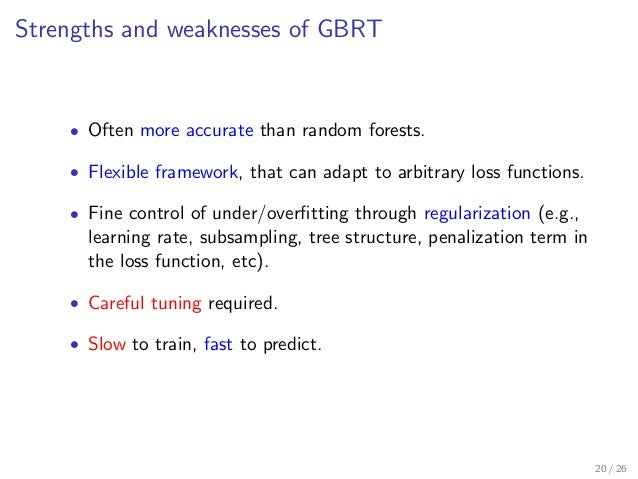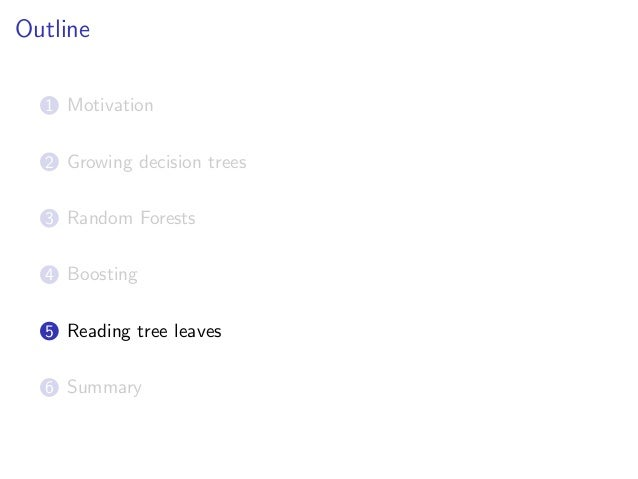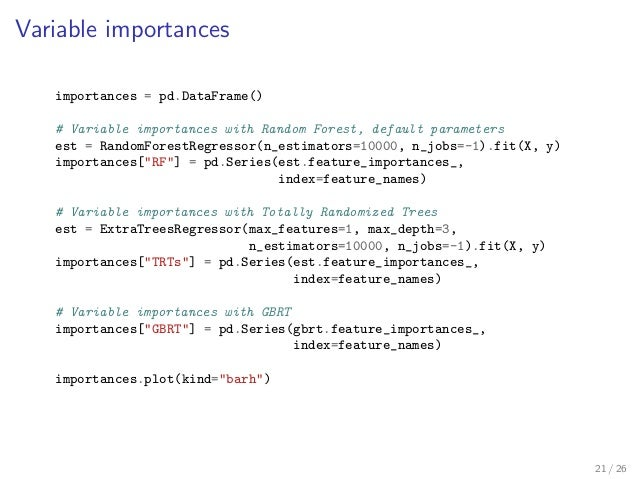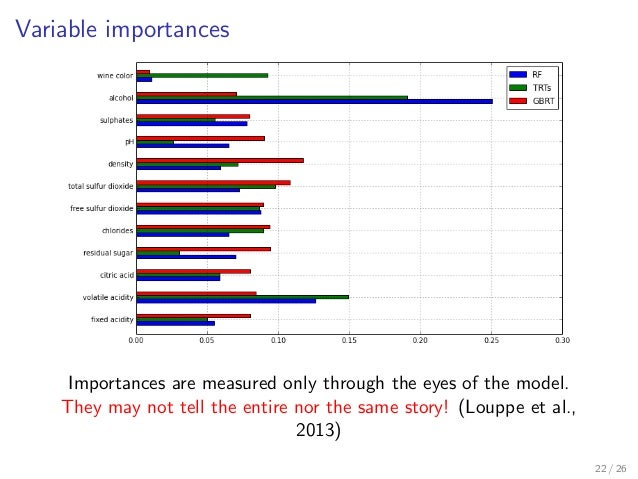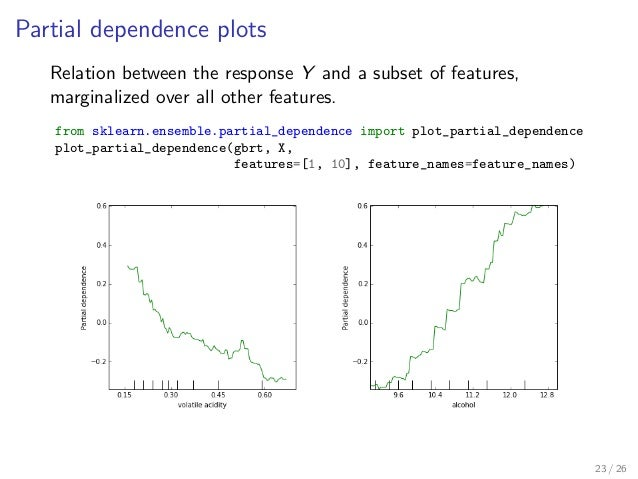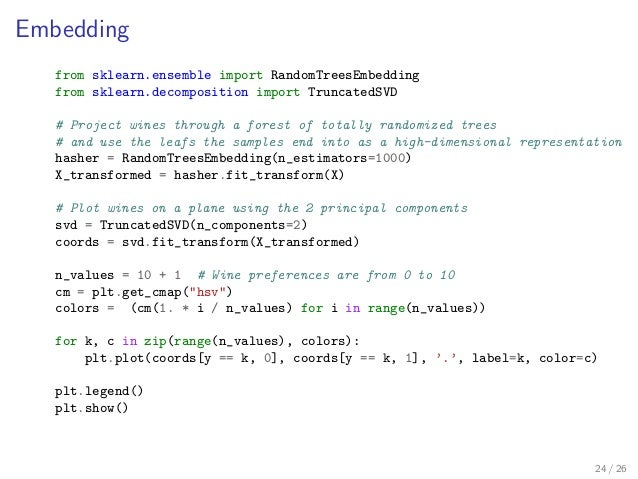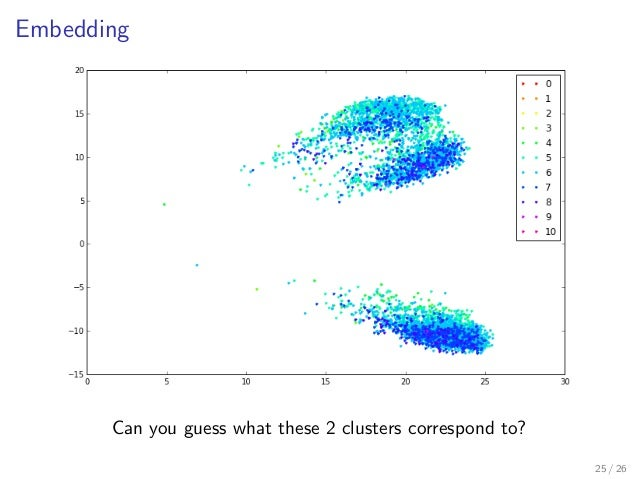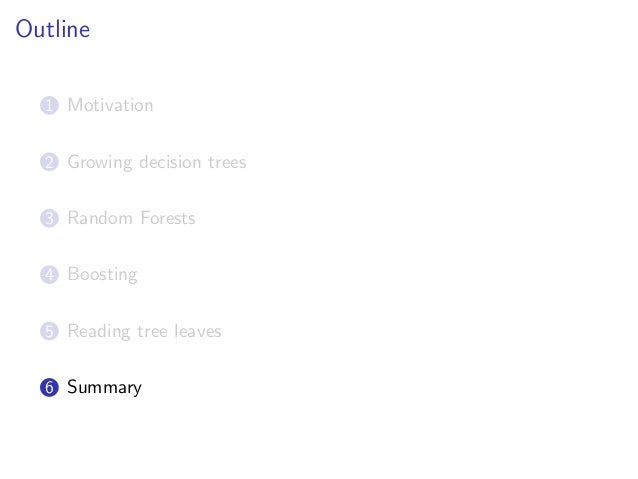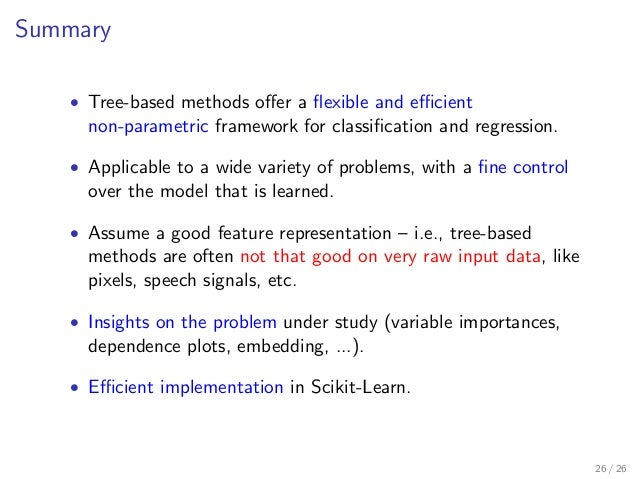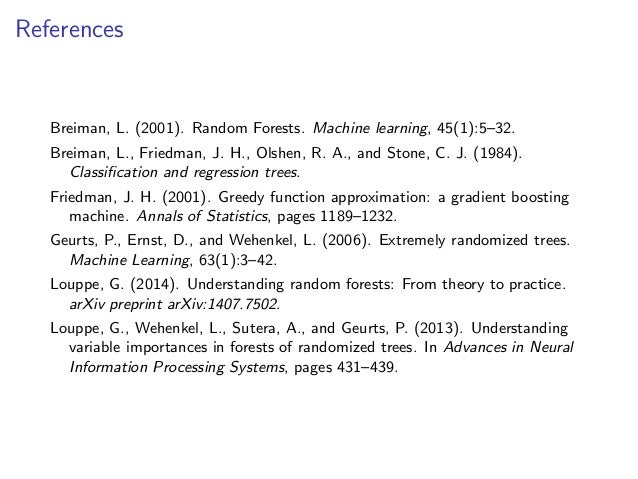Upcoming SlideShare
×

# Tree models with Scikit-Learn: Great models with little assumptions

This talk gives an introduction to tree-based methods, both from a theoretical and practical point of view. It covers decision trees, random forests and boosting estimators, along with concrete examples based on Scikit-Learn about how they work, when they work and why they work.

• Full Name
Comment goes here.

Are you sure you want to Yes No• Be the first to comment

### Tree models with Scikit-Learn: Great models with little assumptions

1. 1. Tree models with Scikit-Learn Great learners with little assumptions Material: https://github.com/glouppe/talk-pydata2015 Gilles Louppe (@glouppe) CERN PyData, April 3, 2015
2. 2. Outline 1 Motivation 2 Growing decision trees 3 Random forests 4 Boosting 5 Reading tree leaves 6 Summary 2 / 26
3. 3. Motivation 3 / 26
4. 4. Running example From physicochemical properties (alcohol, acidity, sulphates, ...), learn a model to predict wine taste preferences. 4 / 26
5. 5. Outline 1 Motivation 2 Growing decision trees 3 Random Forests 4 Boosting 5 Reading tree leaves 6 Summary
6. 6. Supervised learning • Data comes as a ﬁnite learning set L = (X, y) where Input samples are given as an array of shape (n samples, n features) E.g., feature values for wine physicochemical properties: # fixed acidity, volatile acidity, ... X = [[ 7.4 0. ... 0.56 9.4 0. ] [ 7.8 0. ... 0.68 9.8 0. ] ... [ 7.8 0.04 ... 0.65 9.8 0. ]] Output values are given as an array of shape (n samples,) E.g., wine taste preferences (from 0 to 10): y = [5 5 5 ... 6 7 6] • The goal is to build an estimator ϕL : X → Y minimizing Err(ϕL) = EX,Y {L(Y , ϕL.predict(X))}. 5 / 26
7. 7. Decision trees (Breiman et al., 1984) 0.7 0.5 X1 X2 t5 t3 t4 𝑡2 𝑋1 ≤ 0.7 𝑡1 𝑡3 𝑡4 𝑡5 𝒙 𝑝(𝑌 = 𝑐|𝑋 = 𝒙) Split node Leaf node≤ > 𝑋2 ≤ 0.5 ≤ > function BuildDecisionTree(L) Create node t if the stopping criterion is met for t then Assign a model to yt else Find the split on L that maximizes impurity decrease s∗ = arg max s i(t) − pLi(ts L) − pR i(ts R ) Partition L into LtL ∪ LtR according to s∗ tL = BuildDecisionTree(LtL ) tR = BuildDecisionTree(LtR ) end if return t end function 6 / 26
8. 8. Composability of decision trees Decision trees can be used to solve several machine learning tasks by swapping the impurity and leaf model functions: 0-1 loss (classiﬁcation) yt = arg maxc∈Y p(c|t), i(t) = entropy(t) or i(t) = gini(t) Mean squared error (regression) yt = mean(y|t), i(t) = 1 Nt x,y∈Lt (y − yt)2 Least absolute deviance (regression) yt = median(y|t), i(t) = 1 Nt x,y∈Lt |y − yt| Density estimation yt = N(µt, Σt), i(t) = diﬀerential entropy(t) 7 / 26
9. 9. sklearn.tree # Fit a decision tree from sklearn.tree import DecisionTreeRegressor estimator = DecisionTreeRegressor(criterion="mse", # Set i(t) function max_leaf_nodes=5) # Tune model complexity # with max_leaf_nodes, # max_depth or # min_samples_split estimator.fit(X_train, y_train) # Predict target values y_pred = estimator.predict(X_test) # MSE on test data from sklearn.metrics import mean_squared_error score = mean_squared_error(y_test, y_pred) >>> 0.572049826453 8 / 26
10. 10. Visualize and interpret # Display tree from sklearn.tree import export_graphviz export_graphviz(estimator, out_file="tree.dot", feature_names=feature_names) 9 / 26
11. 11. Strengths and weaknesses of decision trees • Non-parametric model, proved to be consistent. • Support heterogeneous data (continuous, ordered or categorical variables). • Flexibility in loss functions (but choice is limited). • Fast to train, fast to predict. In the average case, complexity of training is Θ(pN log2 N). • Easily interpretable. • Low bias, but usually high variance Solution: Combine the predictions of several randomized trees into a single model. 10 / 26
12. 12. Outline 1 Motivation 2 Growing decision trees 3 Random Forests 4 Boosting 5 Reading tree leaves 6 Summary
13. 13. Random Forests (Breiman, 2001; Geurts et al., 2006) 𝒙 𝑝 𝜑1 (𝑌 = 𝑐|𝑋 = 𝒙) 𝜑1 𝜑 𝑀 … 𝑝 𝜑 𝑚 (𝑌 = 𝑐|𝑋 = 𝒙) ∑ 𝑝 𝜓(𝑌 = 𝑐|𝑋 = 𝒙) Randomization • Bootstrap samples } Random Forests• Random selection of K p split variables } Extra-Trees• Random selection of the threshold 11 / 26
14. 14. Bias and variance 12 / 26
15. 15. Bias-variance decomposition Theorem. For the squared error loss, the bias-variance decomposition of the expected generalization error EL{Err(ψL,θ1,...,θM (x))} at X = x of an ensemble of M randomized models ϕL,θm is EL{Err(ψL,θ1,...,θM (x))} = noise(x) + bias2 (x) + var(x), where noise(x) = Err(ϕB(x)), bias2 (x) = (ϕB(x) − EL,θ{ϕL,θ(x)})2 , var(x) = ρ(x)σ2 L,θ(x) + 1 − ρ(x) M σ2 L,θ(x). and where ρ(x) is the Pearson correlation coeﬃcient between the predictions of two randomized trees built on the same learning set. 13 / 26
16. 16. Diagnosing the error of random forests (Louppe, 2014) • Bias: Identical to the bias of a single randomized tree. • Variance: var(x) = ρ(x)σ2 L,θ(x) + 1−ρ(x) M σ2 L,θ(x) As M → ∞, var(x) → ρ(x)σ2 L,θ(x) The stronger the randomization, ρ(x) → 0, var(x) → 0. The weaker the randomization, ρ(x) → 1, var(x) → σ2 L,θ(x) Bias-variance trade-oﬀ. Randomization increases bias but makes it possible to reduce the variance of the corresponding ensemble model. The crux of the problem is to ﬁnd the right trade-oﬀ. 14 / 26
17. 17. Tuning randomization in sklearn.ensemble from sklearn.ensemble import RandomForestRegressor, ExtraTreesRegressor from sklearn.cross_validation import ShuffleSplit from sklearn.learning_curve import validation_curve # Validation of max_features, controlling randomness in forests param_name = "max_features" param_range = range(1, X.shape+1) for Forest, color, label in [(RandomForestRegressor, "g", "RF"), (ExtraTreesRegressor, "r", "ETs")]: _, test_scores = validation_curve( Forest(n_estimators=100, n_jobs=-1), X, y, cv=ShuffleSplit(n=len(X), n_iter=10, test_size=0.25), param_name=param_name, param_range=param_range, scoring="mean_squared_error") test_scores_mean = np.mean(-test_scores, axis=1) plt.plot(param_range, test_scores_mean, label=label, color=color) plt.xlabel(param_name) plt.xlim(1, max(param_range)) plt.ylabel("MSE") plt.legend(loc="best") plt.show() 15 / 26
18. 18. Tuning randomization in sklearn.ensemble Best-tradeoﬀ: ExtraTrees, for max features=6. 16 / 26
19. 19. Strengths and weaknesses of forests • One of the best oﬀ-the-self learning algorithm, requiring almost no tuning. • Fine control of bias and variance through averaging and randomization, resulting in better performance. • Moderately fast to train and to predict. Θ(MKN log2 N) for RFs (where N = 0.632N) Θ(MKN log N) for ETs • Embarrassingly parallel (use n jobs). • Less interpretable than decision trees. 17 / 26
20. 20. Outline 1 Motivation 2 Growing decision trees 3 Random Forests 4 Boosting 5 Reading tree leaves 6 Summary
21. 21. Gradient Boosted Regression Trees (Friedman, 2001) • GBRT ﬁts an additive model of the form ϕ(x) = M m=1 γmhm(x) • The ensemble is built in a forward stagewise manner, where each regression tree hm is an approximate successive gradient step. 2 6 10 x 2.0 1.5 1.0 0.5 0.0 0.5 1.0 1.5 2.0 2.5 y Ground truth 2 6 10 x ∼ tree 1 2 6 10 x + tree 2 2 6 10 x + tree 3 18 / 26
22. 22. Careful tuning required from sklearn.ensemble import GradientBoostingRegressor from sklearn.cross_validation import ShuffleSplit from sklearn.grid_search import GridSearchCV # Careful tuning is required to obtained good results param_grid = {"learning_rate": [0.1, 0.01, 0.001], "subsample": [1.0, 0.9, 0.8], "max_depth": [3, 5, 7], "min_samples_leaf": [1, 3, 5]} est = GradientBoostingRegressor(n_estimators=1000) grid = GridSearchCV(est, param_grid, cv=ShuffleSplit(n=len(X), n_iter=10, test_size=0.25), scoring="mean_squared_error", n_jobs=-1).fit(X, y) gbrt = grid.best_estimator_ See our PyData 2014 tutorial for further guidance https://github.com/pprett/pydata-gbrt-tutorial 19 / 26
23. 23. Strengths and weaknesses of GBRT • Often more accurate than random forests. • Flexible framework, that can adapt to arbitrary loss functions. • Fine control of under/overﬁtting through regularization (e.g., learning rate, subsampling, tree structure, penalization term in the loss function, etc). • Careful tuning required. • Slow to train, fast to predict. 20 / 26
24. 24. Outline 1 Motivation 2 Growing decision trees 3 Random Forests 4 Boosting 5 Reading tree leaves 6 Summary
25. 25. Variable importances importances = pd.DataFrame() # Variable importances with Random Forest, default parameters est = RandomForestRegressor(n_estimators=10000, n_jobs=-1).fit(X, y) importances["RF"] = pd.Series(est.feature_importances_, index=feature_names) # Variable importances with Totally Randomized Trees est = ExtraTreesRegressor(max_features=1, max_depth=3, n_estimators=10000, n_jobs=-1).fit(X, y) importances["TRTs"] = pd.Series(est.feature_importances_, index=feature_names) # Variable importances with GBRT importances["GBRT"] = pd.Series(gbrt.feature_importances_, index=feature_names) importances.plot(kind="barh") 21 / 26
26. 26. Variable importances Importances are measured only through the eyes of the model. They may not tell the entire nor the same story! (Louppe et al., 2013) 22 / 26
27. 27. Partial dependence plots Relation between the response Y and a subset of features, marginalized over all other features. from sklearn.ensemble.partial_dependence import plot_partial_dependence plot_partial_dependence(gbrt, X, features=[1, 10], feature_names=feature_names) 23 / 26
28. 28. Embedding from sklearn.ensemble import RandomTreesEmbedding from sklearn.decomposition import TruncatedSVD # Project wines through a forest of totally randomized trees # and use the leafs the samples end into as a high-dimensional representation hasher = RandomTreesEmbedding(n_estimators=1000) X_transformed = hasher.fit_transform(X) # Plot wines on a plane using the 2 principal components svd = TruncatedSVD(n_components=2) coords = svd.fit_transform(X_transformed) n_values = 10 + 1 # Wine preferences are from 0 to 10 cm = plt.get_cmap("hsv") colors = (cm(1. * i / n_values) for i in range(n_values)) for k, c in zip(range(n_values), colors): plt.plot(coords[y == k, 0], coords[y == k, 1], ’.’, label=k, color=c) plt.legend() plt.show() 24 / 26
29. 29. Embedding Can you guess what these 2 clusters correspond to? 25 / 26
30. 30. Outline 1 Motivation 2 Growing decision trees 3 Random Forests 4 Boosting 5 Reading tree leaves 6 Summary
31. 31. Summary • Tree-based methods oﬀer a ﬂexible and eﬃcient non-parametric framework for classiﬁcation and regression. • Applicable to a wide variety of problems, with a ﬁne control over the model that is learned. • Assume a good feature representation – i.e., tree-based methods are often not that good on very raw input data, like pixels, speech signals, etc. • Insights on the problem under study (variable importances, dependence plots, embedding, ...). • Eﬃcient implementation in Scikit-Learn. 26 / 26
33. 33. References Breiman, L. (2001). Random Forests. Machine learning, 45(1):5–32. Breiman, L., Friedman, J. H., Olshen, R. A., and Stone, C. J. (1984). Classiﬁcation and regression trees. Friedman, J. H. (2001). Greedy function approximation: a gradient boosting machine. Annals of Statistics, pages 1189–1232. Geurts, P., Ernst, D., and Wehenkel, L. (2006). Extremely randomized trees. Machine Learning, 63(1):3–42. Louppe, G. (2014). Understanding random forests: From theory to practice. arXiv preprint arXiv:1407.7502. Louppe, G., Wehenkel, L., Sutera, A., and Geurts, P. (2013). Understanding variable importances in forests of randomized trees. In Advances in Neural Information Processing Systems, pages 431–439.

### Be the first to comment

•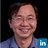#### wengyan

Jun. 11, 2016
•#### JufengJamesPeng

Nov. 17, 2016
•#### happy980

Mar. 14, 2017
•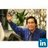#### mikepham12

Apr. 26, 2017

Total views

7,371

On Slideshare

0

From embeds

0

Number of embeds

39

142

Shares

0